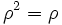# Quasicharacteristic retract

This article defines a subgroup property: a property that can be evaluated to true/false given a group and a subgroup thereof, invariant under subgroup equivalence. View a complete list of subgroup properties[SHOW MORE]

BEWARE! This term is nonstandard and is being used locally within the wiki. [SHOW MORE]

## Definition

### Symbol-free definition

A subgroup of a group is termed a quasicharacteristic retract if there is a retraction (viz, an idempotent endomorphism) on the whole group, whose image is precisely that subgroup) if the following holds:

Any automorphism of the subgroup that extends to an automorphism of the whole group, also pushes forward to an inner automorphism of the automorphism group of the kernel, under the natural map to that automorphism group via the action on the kernel.

### Definition with symbols

Let$H$ be a subgroup of a group$G$.$H$ is termed a quasicharacteristic retract of$G$ if there exists an endomorphism$\rho$ of$G$ such that$\rho^2 = \rho$ and$\rho(G) = H$, and further, if$N$ is the kernel of$\rho$:

Let$\phi: H \to Aut(N)$ be the map sending$h \in H$ to the conjugation map by$h$. Then, for any$\sigma \in Aut(H)$ that extends to an automorphism of$G$, there exists$\sigma'$ an inner automorphism of$Aut(N)$ such that:$\phi \circ \sigma = \sigma' \circ \phi$

## Facts

By the automorphism group action lemma, every characteristic retract is a quasicharacteristic retract.